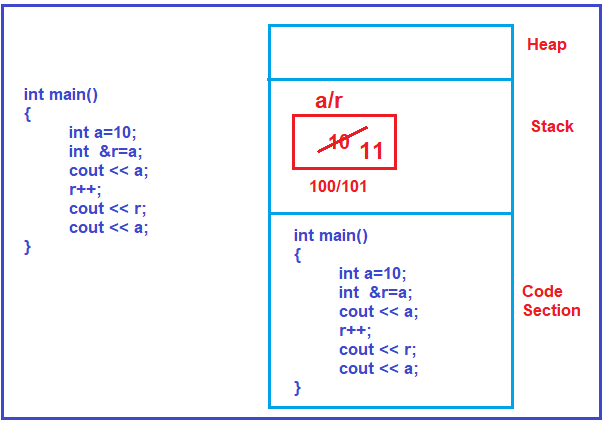# References in C++

## References in C++ | Why should we learn References

In this article, I am going to discuss the basics of References in C++ which is required to learn data structure and algorithm. Please read our previous article where we discussed the basics of Pointers.

##### What Are References?

The reference feature is available only in C++ and this is not available in C language. So, let us understand the Reference. A Reference is a nickname or alias given to a variable.

##### How to make a variable as a reference variable in C++?

When we write & before the variable name in C++, then it is considered as a reference variable. For better understanding, please have a look at the following image.As you can see in the above image, first we declare a data variable i.e. a, and initialized it with the value 10. This variable “a” is created inside the Stack area of the Main memory. We created the variable r with &, so it is a reference variable.

The reference variable must be initialized when declared. Here we assigned the variable “a” i.e. 10 to the reference variable. Now both the variable a and r pointing to the same memory location as what you can see in the image. So, now you can access the memory location using both a and r. In order to prove this, please execute the below code using any C++ editor and observe the output.

```#include <iostream>
using namespace std;

int main ()
{
int a = 10;
int &r = a;
cout << a;
r++;
cout << r;
cout << a;
}
```

So, the reference is nothing but another name to a variable.

##### Why do you need another name for the same variable?

Now the question that should come to your mind is, why do we need another name to the same variable as we can access the same variable using one name. This is used in parameter passing and this is a very useful feature of C++ for writing small functions. We will use references instead of using pointers.

So, when we learn about parameter passing, at that time we will explain what is the use of reference. Right now, in our example, this is a simple main program, in this, we don’t need any reference because already we have ‘a’ we can use ‘a’ but for explaining what a reference is we have used a simple code. So, Reference is nothing but another name given to a variable.

In the next article, I am going to discuss Pointers to Structure which is very important to understand data structure and algorithm. Here, in this article, I try to explain the basics of References in C++ and I hope you enjoy this Reference in C++ article.